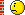Tech Log The very best in practical technical discussion on the web

Temperature Corrections. High Temperature.

Join Date: Jan 2007
Location: Djakarta
Age: 31
Posts: 77
Temperature Corrections. High Temperature.

Hi Just a quick question.
I have temperature corrections for cold weather. However if for instance I was flying an ILS and say the OAT was 38C, the OM crossing height on the chart was say 1200ft and the airport was at MSL, how can I check the Outer Marker height, allowing for the high temperature?Join Date: Aug 2009
Location: Seat 0A
Posts: 516
Since the cold temperature correction is mainly supposed to guarantee your obstacle clearance a higher temperature than ISA would be considered an error on the "safe side"... Therefore you should not correct check heights for higher temperatures. If you still want to do it the temperature error is about 4ft in 1000 ft of height above the altimeter reference per KelvinJoin Date: Jan 2007
Location: Djakarta
Age: 31
Posts: 77
High Temps

STBYRUD

What I should have said is what would the altimeter read in the scenario that I put forward. So in this case the altimeter won't read 1200ft, so what should it read. There must be a rule of thumb to cater for the higher temperature, apart from using the Kelvin Scale is there anything relating to Celsius?

Thanks

arrowJoin Date: Jan 2004
Location: Here and there
Posts: 2,759
The correction is the same whether it is colder or hotter than ISA, 4% change in altitude for every 10ºC deviation from ISA. In ISA+ temps the altimeter will under-read (safe error) and in ISA- temps the altimeter will over-read (unsafe error).

In your scenario the temp is ISA+23.
4% for every 10º deviation so,
4 * 2.3 = 9.2

So if you're on slope at the OM the altimeter should read 9% less than the published height or about 1090'

To make things a bit simpler use 5% for every 10º deviation or 10% for 20º deviation. and round to the nearest 10. That would give you a correction of 120' in your example.Join Date: Mar 2007
Location: Cardiff UK
Age: 65
Posts: 118
By definition the magnitude of one degree Celsius and one degree Kelvin are exactly the same. Zero degree Kelvin is absolute zero, which is also minus 273.15 degrees Celsius and therefore zero degrees Celsius is 273.15 degrees Kelvin.Join Date: Oct 2009
Location: UK
Posts: 1,271
Hi arrow28,

Just to follow on from Nick Thomas' comment & AerocatS2A's maths: -
With your temperature deviation from ISA in degs C,
divide by 288 (273+15)
and multiply by the Altitude,
that's the correction to be added / subtracted.Join Date: Jan 2007
Location: Djakarta
Age: 31
Posts: 77
Altitude Correction

How would the calculation run if say the airfield was 6000ft above MSL, the OAT was 35*C and the ILS check altitude was 7200ft. What should the altimeter read.?

Thanks

arrow.Join Date: Aug 1998
Location: Ex-pat Aussie in the UK
Posts: 4,542
How would the calculation run if say the airfield was 6000ft above MSL, the OAT was 35*C and the ILS check altitude was 7200ft. What should the altimeter read.?
First step: Work out your ISA deviation.
Second step: multiply that by 4 (double it, then double it again)
Third step: multiply the second step number by the height above the field (in thousands of feet).
Fourth step: temperature deviation LOW, look out beLOW (i.e. LOW temperatures mean you are closer to the ground than indicated, conversely high temperatures are "safer"). If the ISA deviation was negative, ADD the number to the true height off the chart to find your altimeter reading at that height.

For the above particular problem:
1. At 6000' AMSL the ISA temp should be 15ºC-(6000' times 2º/1000') = 15-12 = 3ºC. Reported temp is 35ºC, so you are ISA+32º
2. 32 doubled is 64, doubled is 128
3. Check altitude is 7,200' so check height is 1,200'. 128 times 1.2 (thousands of feet) = 153.6 (154 feet rounded up)
4. Temp is high, so subtract the 154 from the crossing height :~ 7,200' - 154 = 7,046 feet. That is what your altimeter will read passing the ILS check height.

So, if the altitude of 7,200 was your MDA, and you went around at 7,200 indicated - you would have gone around 154 feet too high - safer, because it's hotter.Join Date: Apr 2011
Location: NL
Age: 34
Posts: 93
Sorry for the stupid mistake before.I totally forgot about only using the real-air part (height) like checkerboard said.

Using 4% for 10 degrees deviation from isa you get: 34 degrees warmer than standard on 1200 feet height, This makes true altitude about 14 % higher than indicated. So 14% of 1200 feet is 168 feet.

This is close to Checkerboard's answer so I hope it is correct now.You may not post new threads
You may not post replies
You may not post attachments
You may not edit your posts

BB code is On
Smilies are On
[IMG] code is On
HTML code is OffShow Printable VersionEmail this Page